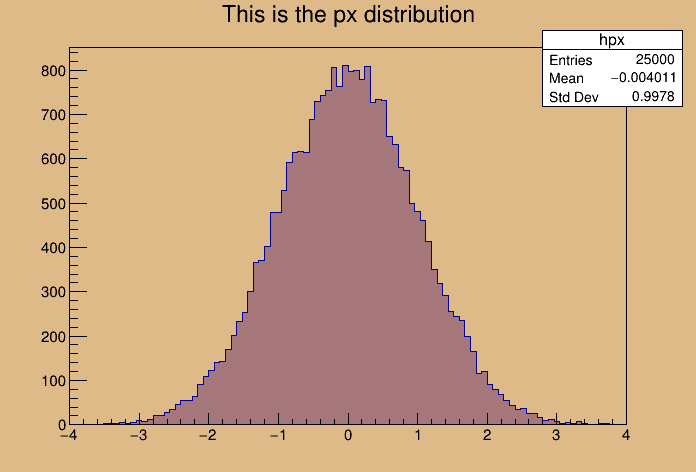# Hsimple¶

This program creates :

• a one dimensional histogram
• a two dimensional histogram
• a profile histogram
• a memory-resident ntuple

These objects are filled with some random numbers and saved on a file. If get=1 the macro returns a pointer to the TFile of "hsimple.root"

if this file exists, otherwise it is created.


The file "hsimple.root" is created in $ROOTSYS/tutorials if the caller has write access to this directory, otherwise the file is created in$PWD

Author: Rene Brun
This notebook tutorial was automatically generated with ROOTBOOK-izer from the macro found in the ROOT repository on Monday, January 27, 2020 at 02:07 AM.

In :
%%cpp -d
#include <TFile.h>
#include <TNtuple.h>
#include <TH2.h>
#include <TProfile.h>
#include <TCanvas.h>
#include <TFrame.h>
#include <TROOT.h>
#include <TSystem.h>
#include <TRandom3.h>
#include <TBenchmark.h>
#include <TInterpreter.h>


Arguments are defined.

In :
Int_t getFile=0;

In :
TString filename = "hsimple.root";
TString dir = gROOT->GetTutorialDir();
dir.ReplaceAll("/./","/");
TFile *hfile = 0;
if (getFile) {
// if the argument getFile =1 return the file "hsimple.root"
// if the file does not exist, it is created
TString fullPath = dir+"hsimple.root";
if (!gSystem->AccessPathName(fullPath,kFileExists)) {
hfile = TFile::Open(fullPath); //in $ROOTSYS/tutorials if (hfile) return hfile; } //otherwise try$PWD/hsimple.root
if (!gSystem->AccessPathName("hsimple.root",kFileExists)) {
hfile = TFile::Open("hsimple.root"); //in current dir
if (hfile) return hfile;
}
}


No hsimple.root file found. must generate it ! generate hsimple.root in current directory if we have write access

In :
if (gSystem->AccessPathName(".",kWritePermission)) {
printf("you must run the script in a directory with write access\n");
return 0;
}
hfile = (TFile*)gROOT->FindObject(filename); if (hfile) hfile->Close();
hfile = new TFile(filename,"RECREATE","Demo ROOT file with histograms");


Create some histograms, a profile histogram and an ntuple

In :
TH1F *hpx = new TH1F("hpx","This is the px distribution",100,-4,4);
hpx->SetFillColor(48);
TH2F *hpxpy = new TH2F("hpxpy","py vs px",40,-4,4,40,-4,4);
TProfile *hprof = new TProfile("hprof","Profile of pz versus px",100,-4,4,0,20);
TNtuple *ntuple = new TNtuple("ntuple","Demo ntuple","px:py:pz:random:i");

gBenchmark->Start("hsimple");


Create a new canvas.

In :
TCanvas *c1 = new TCanvas("c1","Dynamic Filling Example",200,10,700,500);
c1->SetFillColor(42);
c1->GetFrame()->SetFillColor(21);
c1->GetFrame()->SetBorderSize(6);
c1->GetFrame()->SetBorderMode(-1);


Fill histograms randomly

In :
TRandom3 randomNum;
Float_t px, py, pz;
const Int_t kUPDATE = 1000;
for (Int_t i = 0; i < 25000; i++) {
randomNum.Rannor(px,py);
pz = px*px + py*py;
Float_t rnd = randomNum.Rndm();
hpx->Fill(px);
hpxpy->Fill(px,py);
hprof->Fill(px,pz);
ntuple->Fill(px,py,pz,rnd,i);
if (i && (i%kUPDATE) == 0) {
if (i == kUPDATE) hpx->Draw();
c1->Modified();
c1->Update();
if (gSystem->ProcessEvents())
break;
}
}
gBenchmark->Show("hsimple");

hsimple   : Real Time =   0.30 seconds Cpu Time =   0.18 seconds


Save all objects in this file

In :
hpx->SetFillColor(0);
hfile->Write();
hpx->SetFillColor(48);
c1->Modified();
return hfile;


Note that the file is automatically close when application terminates or when the file destructor is called.

Draw all canvases

In :
gROOT->GetListOfCanvases()->Draw()